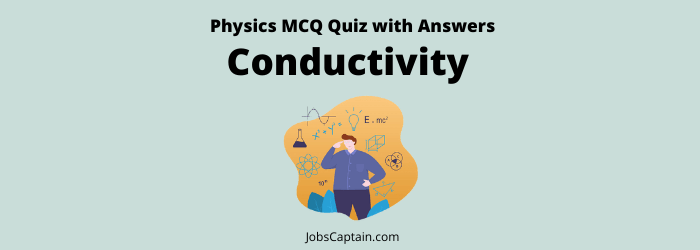# Physics: Conductivity MCQ QuizQuestion 1. At absolute zero temperature, the electric resistance in semiconductor is_______.

(A) Zero

(B) High

(C) Meager

(D) Infinite

(D) Infinite

Question 2. Which one of the following metals is used as semi-conductor in transistors?

(A) Silver

(B) Graphite

(C) Germanium

(D) Copper

(C) Germanium

Question 3. The most commonly used material for making transistors is ________.

(A) Silver

(B) Copper

(C) Silicon

(D) Aluminium

(C) Silicon

Question 4. Which one of the following is an important component of a transistor?

(B) Osmium

(C) Germanium

(D) Arsenic

(C) Germanium

Question 5. Which of the following is a semiconductor?

(A) Germanium

(B) Wood

(C) Aluminium

(D) Plastic

(A) Germanium

Question 6. The Government of India has totally banned the export of Burnt coal (Jhama) because it contains recoverable amount of a metal/ element which is used in transistors. Which one of the following is that element?

(A) Tungsten

(B) Silicon

(C) Germanium

(D) Phosphorus

(C) Germanium

Question 7. The efforts to detect the existence of Higgs Boson particle have become frequent news in the recent past. What is/are the importance of discovering this particle?

1. It will help us to understand as to why elementary particles have mass.
2. It will enable us in the near future to develop the technology to transfer matter from one point to another without traversing the physical space between them.
3. It will enable us to create better fuel for nuclear fission.

Select the correct answer using the codes given below.

(A) All 1, 2 and 3

(B) Only 1 and 3

(C) Only 2 and 3

(D) Only 1

(D) Only 1

Question 8.

• Assertion (A): Copper rods are generally preferred to iron rods for making lightning conductors.
• Reason (R): Copper is better conductor of electricity than iron and is not easily oxidized under atmospheric conditions.

(A) (A) is false, but (R) is true

(B) (A) is true, but (R) is false

(C) Both (A) and (R) are true, but (R) is not the correct explanation of (A)

(D) Both (A) and (R) are true and (R) is the correct explanation of (A)

(D) Both (A) and (R) are true and (R) is the correct explanation of (A)

Question 9. Which of the following are semiconductors?

1. Silicon
2. Quartz
3. Ceramics
4. Germanium

Choose the correct answer from the following alternatives.

(A) 3 and 4

(B) 1 and 4

(C) 1 and 3

(D) 1 and 2

(B) 1 and 4

Question 10. Electric conduction in a semiconductor takes place due to__________.

(A) Neither electrons nor holes

(B) Both electrons and holes

(C) Holes only

(D) Electrons only

(B) Both electrons and holes

Question 11. The resistance of a semi-conductor on heating_______.

(A)Remains constant

(B) Increases

(C) Decreases

(D) None of the above

(C) Decreases

Question 12. The conductivity of a semiconductor at zero degree Kelvin is________.

(A) Zero

(B) 10–5 ohm

(C) 10–1 ohm

(D) 105 ohm

(A) Zero

Question 13. At which temperature superconductivity can be of tremendous economic interest saving billions of rupees?

(A) At a very high temperature

(B) At room temperature

(C) At a temperature when semiconductor becomes a super conductor

(D) At very low temperature

(B) At room temperature

Question 14. The characteristic of super conductor is________.

(A) Infinite permeability

(B) Zero permeability

(C) Low permeability

(D) High permeability

(B) Zero permeability

Question 15. The newly discovered high temperature super conductors are_______.

(A) Inorganic polymers

(B) Ceramic oxides

(C) Pure rare earth metals

(D) Metal alloys

(B) Ceramic oxides

Question 16. The highest temperature attained by a super conductor is________.

(A) 300 K

(B) 150 K

(C) 133 K

(D) 24 K